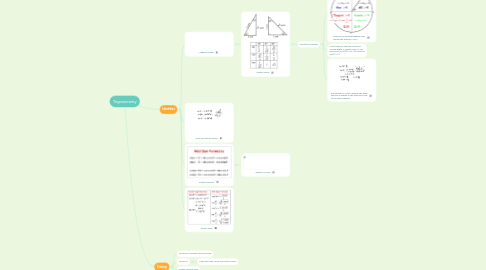# TrigonometryClaudia Foo
Get Started. It's FreeTrigonometry## 1. Identities

### 1.1. Negative Angles

1.1.1. Special Angles

1.1.1.1. Evaluation Questions

1.1.1.1.1. Given info on positive/negative trigo. Identify the quadrant. ACTS

1.1.1.1.2. Use Pythagoras' Theorem to look for missing length. If tangent ratio is a, this means ratio of (o)/(a) is a/1. (h) becomes sqrt(a^2+1)

1.1.1.1.3. Ensure that you insert negative sign when the trigo is applied on the angle not in the first or same quadrant.

## 2. Solving

### 2.2. Find ACTS

2.2.1. Find basic angle, using only positive values